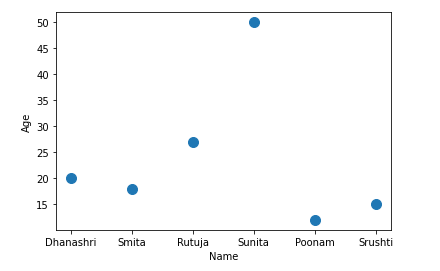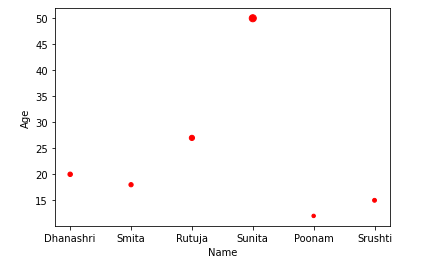# Pandas Scatter Plot – DataFrame.plot.scatter()

• Last Updated : 25 Feb, 2021

A Scatter plot is a type of data visualization technique that shows the relationship between two numerical variables. For plotting to scatter plot using pandas there is DataFrame class and this class has a member called plot. Calling the scatter() method on the plot member draws a plot between two variables or two columns of pandas DataFrame.

Syntax: DataFrame.plot.scatter(x, y, s = none, c = none)

Attention geek! Strengthen your foundations with the Python Programming Foundation Course and learn the basics.

To begin with, your interview preparations Enhance your Data Structures concepts with the Python DS Course. And to begin with your Machine Learning Journey, join the Machine Learning - Basic Level Course

Parameter:

x: column name to be used as horizontal coordinates for each point
y: column name to be used as vertical coordinates for each point
s: size of dots
c: color of dots

### Steps:

• Import necessary libraries.
• Prepare a data
• Convert prepared data into DataFrame
• Draw a scatter plot

Example 1: In this example, we will plot the scatter plot using dataframe, Here we will create the dataframe and plot the scatter plot using different columns.

## Python3

 `# Program to draw scatter plot using Dataframe.plot``# Import libraries``import` `pandas as pd`` ` `# Prepare data``data``=``{``'Name'``:[``'Dhanashri'``, ``'Smita'``, ``'Rutuja'``,``              ``'Sunita'``, ``'Poonam'``, ``'Srushti'``],``      ``'Age'``:[``20``, ``18``, ``27``, ``50``, ``12``, ``15``]}`` ` `# Load data into DataFrame``df ``=` `pd.DataFrame(data ``=` `data);`` ` `# Draw a scatter plot``df.plot.scatter(x ``=` `'Name'``, y ``=` `'Age'``, s ``=` `100``);`

Output:Example 2: Here we will create the dataframe and plot the scatter plot using different columns with style data points.

## Python3

 `# Program to draw scatter plot using Dataframe.plot``# Import libraries``import` `pandas as pd`` ` `# Prepare data``data``=``{``'Name'``:[``'Dhanashri'``, ``'Smita'``, ``'Rutuja'``,``              ``'Sunita'``, ``'Poonam'``, ``'Srushti'``],``      ``'Age'``:[ ``20``, ``18``, ``27``, ``50``, ``12``, ``15``]}`` ` `# Load data into DataFrame``df ``=` `pd.DataFrame(data ``=` `data);`` ` `# Draw a scatter plot and here size of dots determined by age of person``df.plot.scatter(x ``=` `'Name'``, y ``=` `'Age'``, s ``=` `'Age'``, c ``=` `'red'``);`

Output:My Personal Notes arrow_drop_up